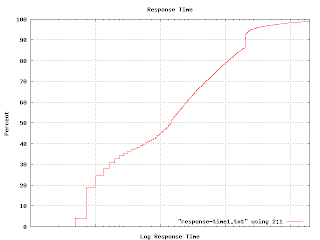## Wednesday, August 22, 2007

Robert W. Baldwin

1957 - 2007

## Saturday, August 18, 2007

### How I do love blogging

So I tried changing the blog settings to not convert line breaks and now all the paragraphs run together. I tried putting in extra blank lines between paragraphs and they disappear, but the first paragraph has extra large spacing between the lines. All these posts look fantastic in the preview mode, but as soon as I hit publish, the web gremlins of ugliness sprinkle their magic style directives on my text.

### Another Couple of GraphsI mentioned in an earlier post that I tried using a cumulative probability graph without much success. It turns out that this sort of graph comes in handy for answering particular kinds of questions. For example, I wanted to illustrate the various response times of one class of machines, so I generated this graph. (The actual graph has numbers along the X axis. Imagine that the tic marks represent `centons' in the middle, `microns' to the left and `arns' to the right.)

The percent axis tells you what percent of response times are faster than the graphed point. That is, to find the median response time, go to the 50% mark and read across and then down. You'll find that the median is a tad over 1 centon. Half of the responses happen faster, half take longer. The 92 percentile is at 1 'arn'. It is pretty unlikely you'd have to wait this long for an answer, but not impossible. Very few responses seem to come in at a `micron'. It turns out that many measurable properties have these S-shaped curves. That isn't surprising because this curve is simply the integral of the underlying distribution (which is often Gaussian). But plotting like this avoids the bucketing issues I was having earlier. If you plot a graph like this and find kinks and non-linearities in it, you have discovered some outliers. This graph shows one.That hook-shaped kink in the upper right is caused by a particular bug which prevented some of the machines from responding until a certain timeout had expired. The timeout value is excessively long and this graph makes that obvious.
Empirically, my observed samples have a log-normal distribution. This is quite interesting because log-normal distributions behave quite differently from Gaussian distributions. For example, the average value falls quite far from the median. The log-normal distribution is also highly asymmetric. You need to use the geometric mean and geometric standard deviation rather than the more common arithmetic mean and standard deviation.

## Thursday, August 9, 2007

### What's missing from this picture?

A friend was conducting an interview the other day and asked the applicant to write a program that traversed a tree in depth-first order. The applicant started by defining a class for the nodes in the tree:
```class Node {
int value;
Node parent;
}

```
Well, it is certainly space efficient. Optimized for going up the tree, too.

## Tuesday, August 7, 2007

### Naive Bayesian Classifier

In analyzing my data I wanted to classify it with a naive Bayesian classifier. I wasn't sure I had the math right, so I wrote a tiny abstract classifier to test with. The code is pretty cool:

```(define (10log10 x)
(* 10.0 (/ (log x) (log 10.0))))
;
(define (sum f list)
(fold-left (lambda (sum element)
(+ sum (f element)))
0
list))
;
(define (count-if predicate list)
(sum (lambda (element)
(if (predicate element)
1
0))
list))
;
(define (count-if-not predicate list)
(sum (lambda (element)
(if (predicate element)
0
1))
list))
;
(define (weight-if-present has-feature? positive-examples
negative-examples)
(10log10
(/ (* (+ (count-if has-feature? positive-examples) .5)
(length negative-examples))
(* (+ (count-if has-feature? negative-examples) .5)
(length positive-examples)))))
;
(define (weight-if-absent has-feature? positive-examples
negative-examples)
(10log10
(/ (* (+ (count-if-not has-feature? positive-examples) .5)
(length negative-examples))
(* (+ (count-if-not has-feature? negative-examples) .5)
(length positive-examples)))))
;
(define (weight has-feature?
positive-examples negative-examples
test-element)
(if (has-feature? test-element)
(weight-if-present has-feature? positive-examples
negative-examples)
(weight-if-absent  has-feature? positive-examples
negative-examples)))
;
(define (naive-bayesian-classifier feature-list positive-examples
negative-examples)
(lambda (test-element)
(sum (lambda (feature)
(weight feature
positive-examples negative-examples
test-element))
feature-list)))
```

.

Obviously this can radically improved for performance. Also note that your version of Scheme might take the arguments to fold-left in a different order. In Statistics and the War on Spam, Dave Madigan gives a really good introduction to naive Bayesian classifiers. The implementation above is based on that paper. The paper also describes why you add .5 to all the counts. To take the example directly from the paper:

```;;; Message sets with stop words removed
;;; and word stemming applied.
(define *ham-messages*
'((brown fox quick)
(rabbit run run run)))
;
(define *spam-messages*
'((quick rabbit run run)
(rabbit rest)))
;
;;; A list of six procedures, each tests for
;;; the presence of a single word in a message.
(define *features*
(map (lambda (word)
(lambda (message)
(member word message)))
'(brown fox quick rabbit rest run)))
;
(define *example-classifier*
(naive-bayesian-classifier
*features*
*ham-messages*
*spam-messages*))
;
;;; Classify a new message.
(*example-classifier* '(quick rabbit rest))
;Value: -11.426675035687316
```

.

The value returned differs from the one in the paper for two reasons. I use positive values to indicate `ham' and negative for `spam', rather than vice-versa. I also compute the logarithm in base 10 rather than the natural log and multiply the result by 10 (decibels rule!). This makes it much easier to eyeball the results. Negative is bad, and every 10 dB is another order of magnitude. With a value of -11, I can see that this is spam with a better than 90% probability. (10 dB = 90%, 20 dB = 99%, 30 dB = 99.9%, 40 dB = 99.99%, etc.) Stupid blogger removes blank lines in PRE sections, so I inserted semicolons. It also has problems transitioning back out of PRE, so I have periods in there as well. I don't like to blog anyway, and this just adds another reason to avoid it.